GAMES101-Lecture03 Transformation

GAMES101-Lecture04 Transformation Cont.

线性变换

$\begin{array}{l}x^{\prime}=a x+b y \\\ y^{\prime}=c x+d y\end{array}$

$\left[\begin{array}{l}x^{\prime} \\\ y^{\prime}\end{array}\right]=\left[\begin{array}{ll}a & b \\\ c & d\end{array}\right]\left[\begin{array}{l}x \\\ y\end{array}\right]$

$\mathbf{x}^{\prime}=\mathbf{M} \mathbf{x}$

Scale Matrix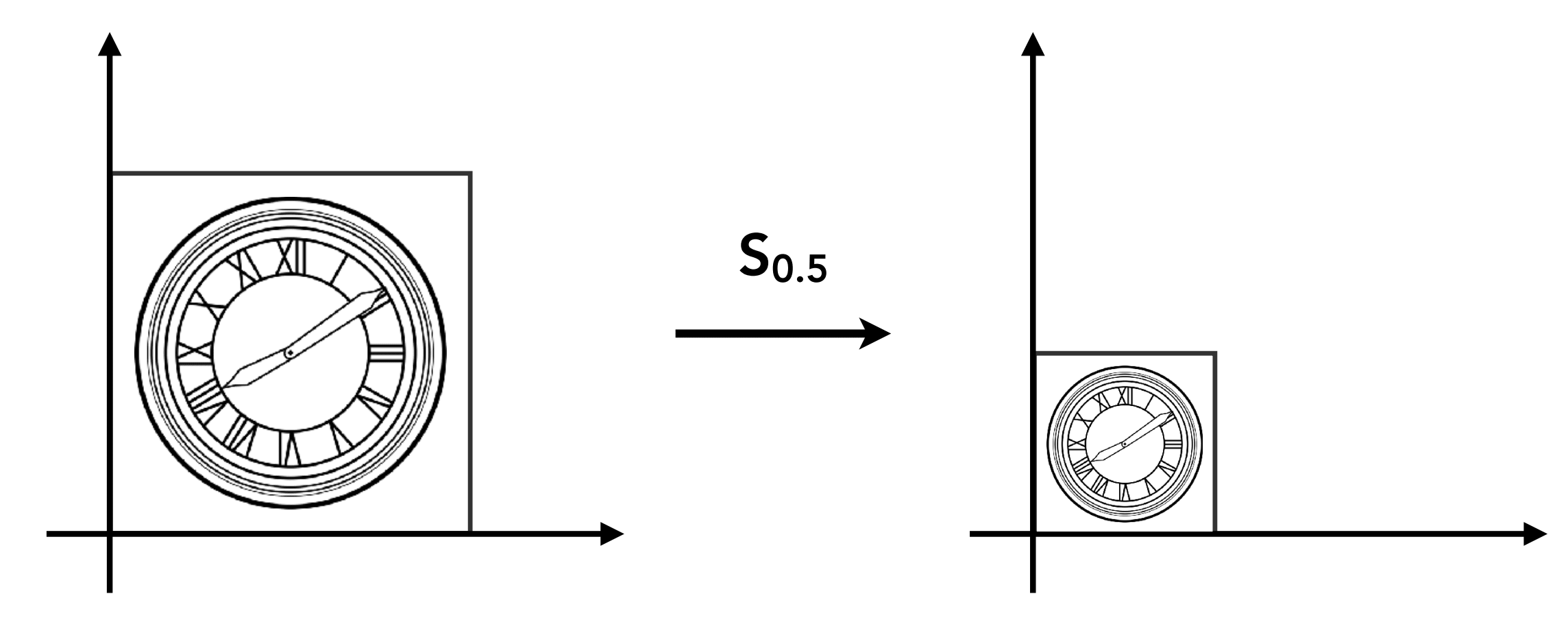$\begin{array}{l}x^{\prime}=s x \\\ y^{\prime}=s y\end{array}$

$\left[\begin{array}{l}x^{\prime} \\\ y^{\prime}\end{array}\right]=\left[\begin{array}{ll}s & 0 \\\ 0 & s\end{array}\right]\left[\begin{array}{l}x \\\ y\end{array}\right]$

Scale (Non-Uniform)

x y 可以不均匀地缩放$\left[\begin{array}{l}x^{\prime} \\\ y^{\prime}\end{array}\right]=\left[\begin{array}{ll}s_{x} & 0 \\\ 0 & s_{y}\end{array}\right]\left[\begin{array}{l}x \\\ y\end{array}\right]$

Reflection Matrix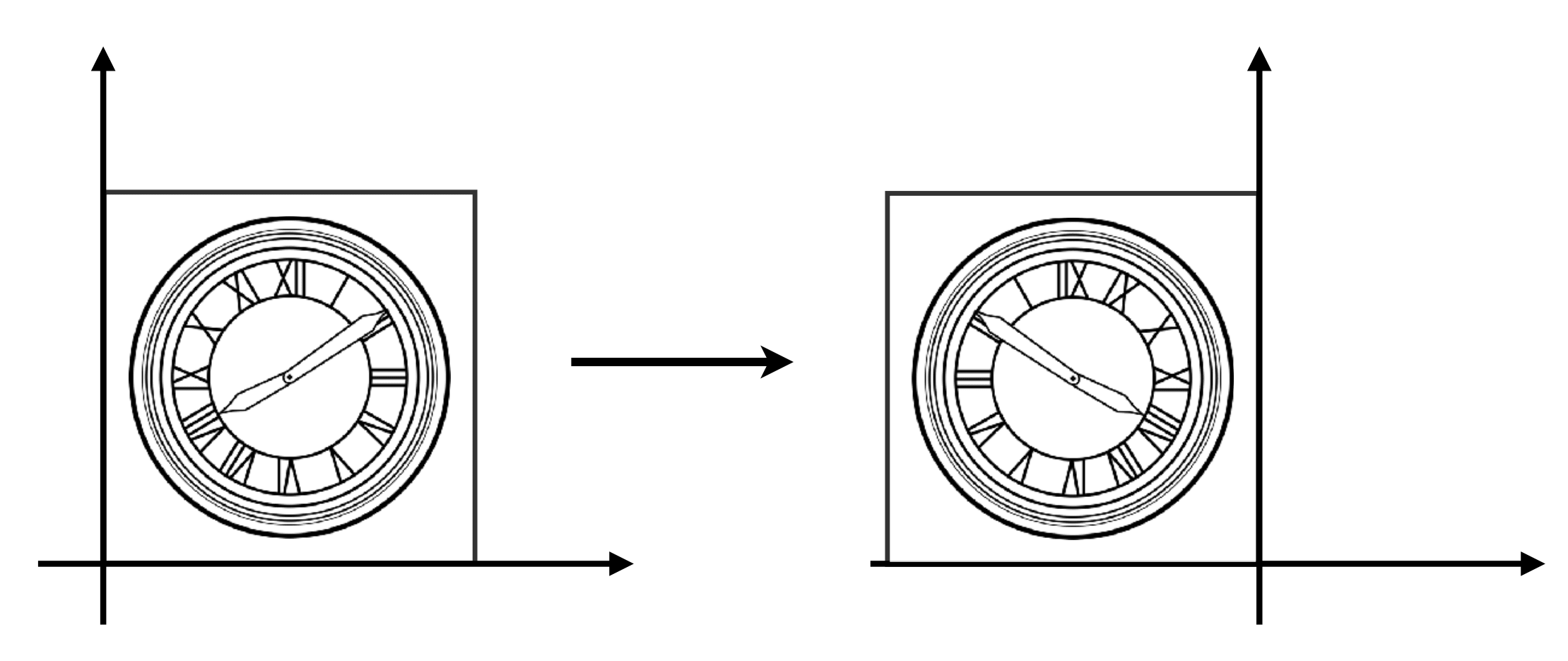Horizontal reflection:

$\begin{array}{l}x^{\prime}=-x \\\ y^{\prime}=y\end{array}$

$\left[\begin{array}{l}x^{\prime} \\\ y^{\prime}\end{array}\right]=\left[\begin{array}{cc}-1 & 0 \\\ 0 & 1\end{array}\right]\left[\begin{array}{l}x \\\ y\end{array}\right]$

Shear Matrix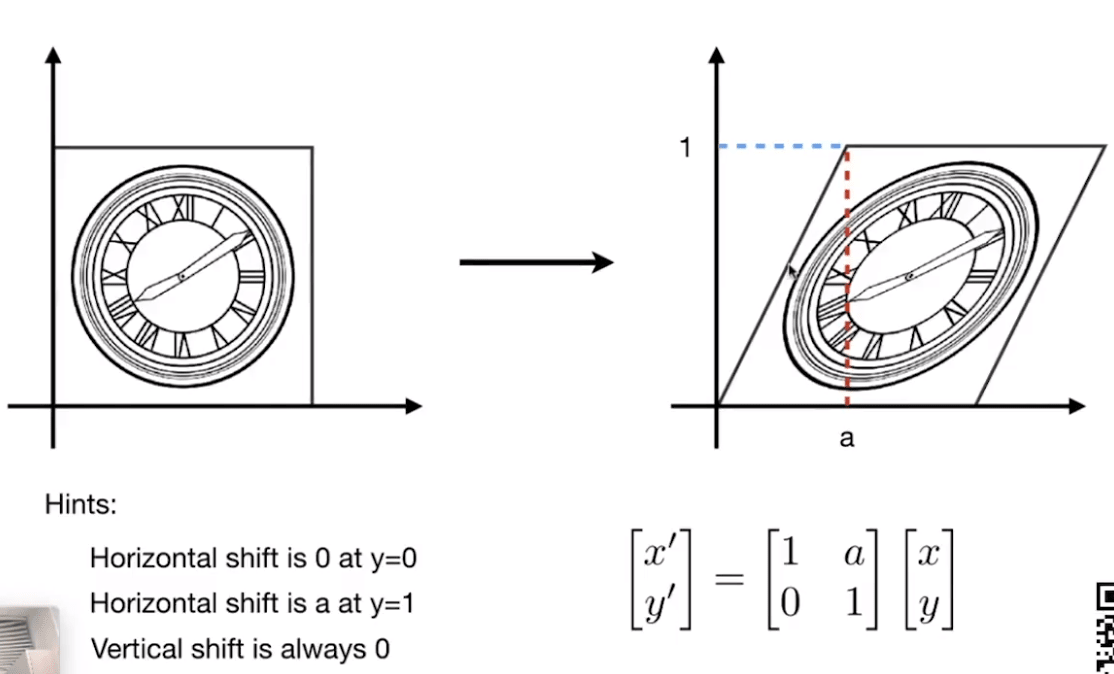2D Rotation Matrix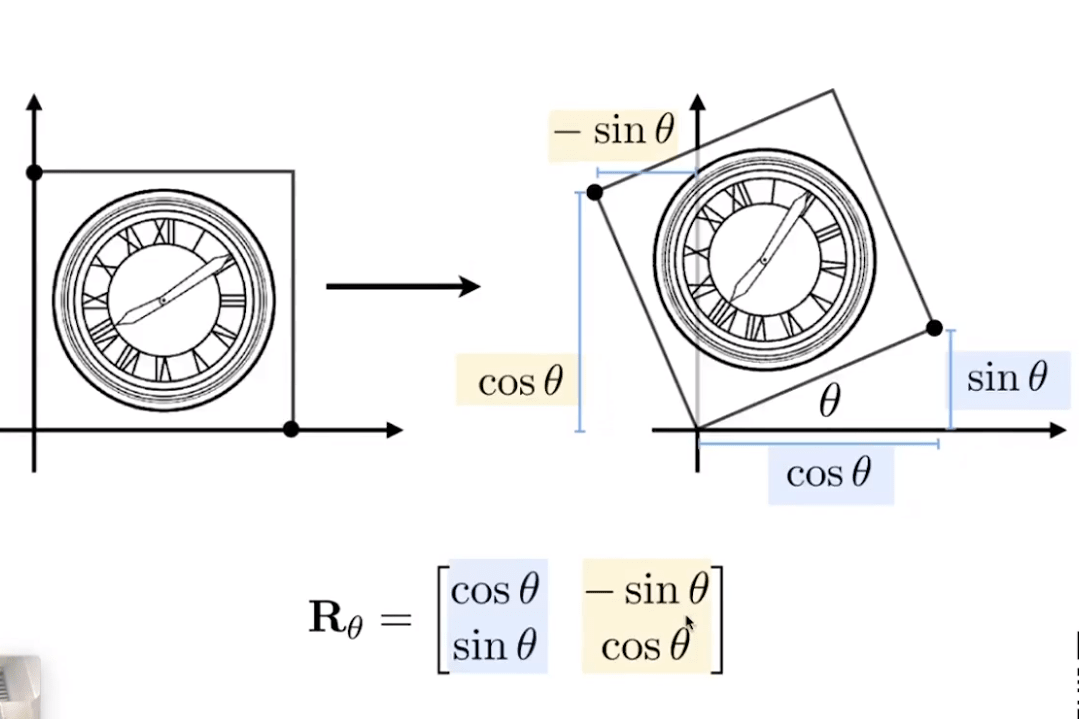齐次坐标

Translation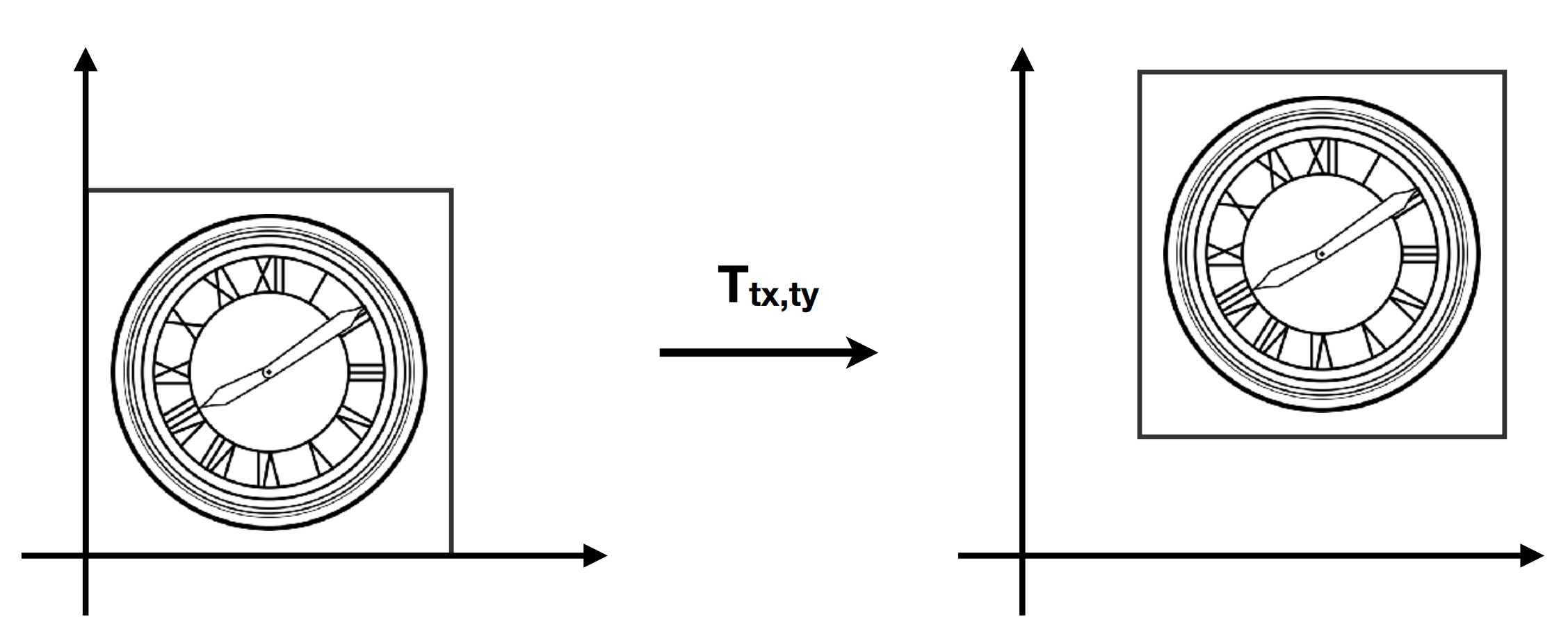$\begin{array}{l}x^{\prime}=x+t_{x} \\\ y^{\prime}=y+t_{y}\end{array}$

$\left[\begin{array}{l}x^{\prime} \\\ y^{\prime}\end{array}\right]=\left[\begin{array}{ll}a & b \\\ c & d\end{array}\right]\left[\begin{array}{l}x \\\ y\end{array}\right]$

$\left[\begin{array}{l}x^{\prime} \\\ y^{\prime}\end{array}\right]=\left[\begin{array}{ll}a & b \\\ c & d\end{array}\right]\left[\begin{array}{l}x \\\ y\end{array}\right]+\left[\begin{array}{l}t_{x} \\\ t_{y}\end{array}\right]$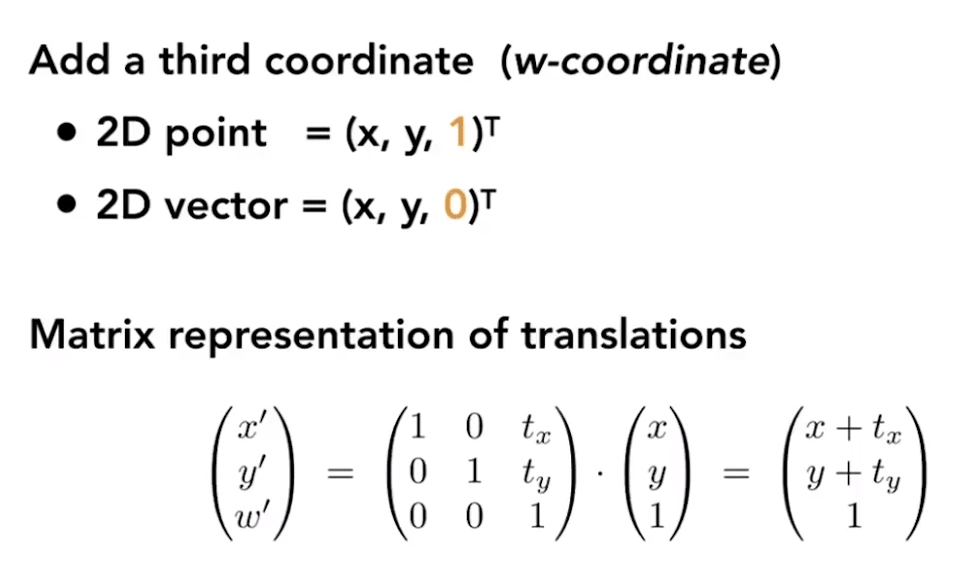Valid operation if w-coordinate of result is 1 or 0

• vector + vector = vector
• point – point = vector
• point + vector = point
• point + point = ??

Affine Transformations 仿射变换

Affine map = linear map + translation

$\left(\begin{array}{l}x^{\prime} \\\ y^{\prime}\end{array}\right)=\left(\begin{array}{ll}a & b \\\ c & d\end{array}\right) \cdot\left(\begin{array}{l}x \\\ y\end{array}\right)+\left(\begin{array}{l}t_{x} \\\ t_{y}\end{array}\right)$

Using homogenous coordinates:

$\left(\begin{array}{l}x^{\prime} \\\ y^{\prime} \\\ 1\end{array}\right)=\left(\begin{array}{ccc}a & b & t_{x} \\\ c & d & t_{y} \\\ 0 & 0 & 1\end{array}\right) \cdot\left(\begin{array}{l}x \\\ y \\\ 1\end{array}\right)$

Scale

$\mathbf{S}\left(s_{x}, s_{y}\right)=\left(\begin{array}{ccc}s_{x} & 0 & 0 \\\ 0 & s_{y} & 0 \\\ 0 & 0 & \text { 1 }\end{array}\right)$

Rotation

$\mathbf{R}(\alpha)=\left(\begin{array}{ccc}\cos \alpha & -\sin \alpha & 0 \\\ \sin \alpha & \cos \alpha & 0 \\\ 0 & 0 & 1\end{array}\right)$

Translation

$\mathbf{T}\left(t_{x}, t_{y}\right)=\left(\begin{array}{ccc}1 & 0 & t_{x} \\\ 0 & 1 & t_{y} \\\ 0 & 0 & 1\end{array}\right)$

逆变换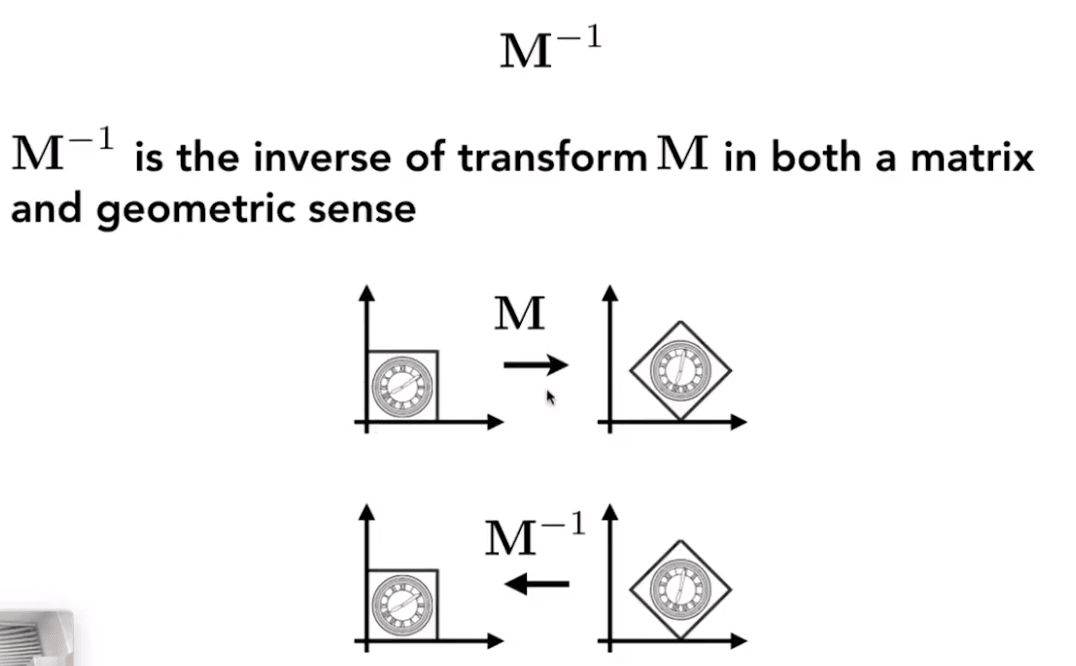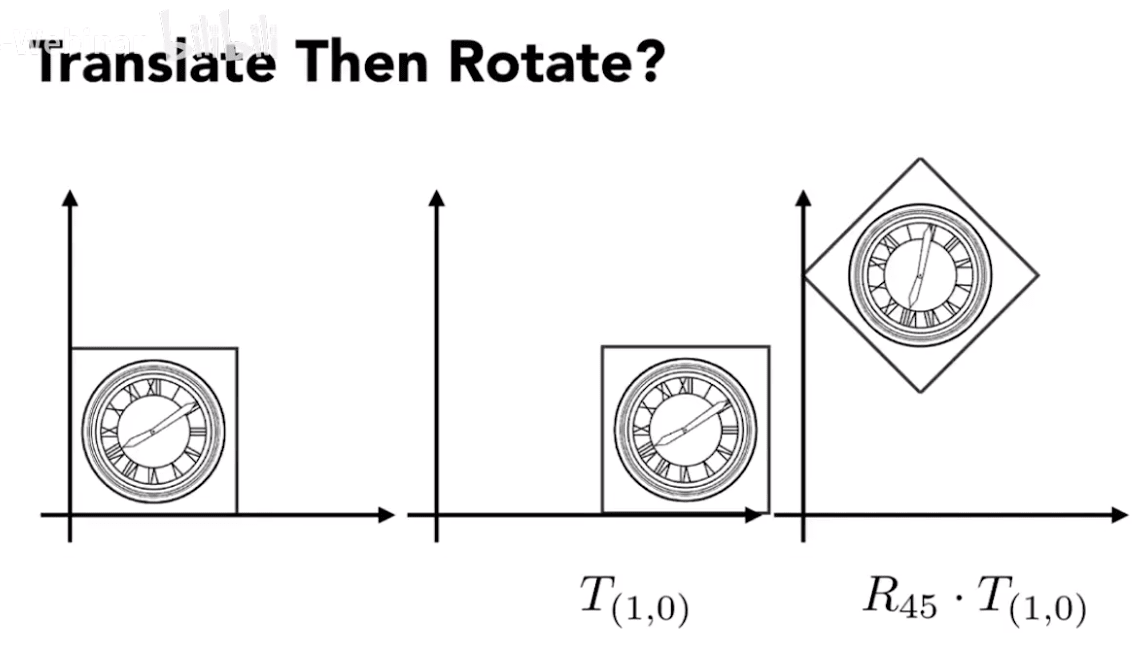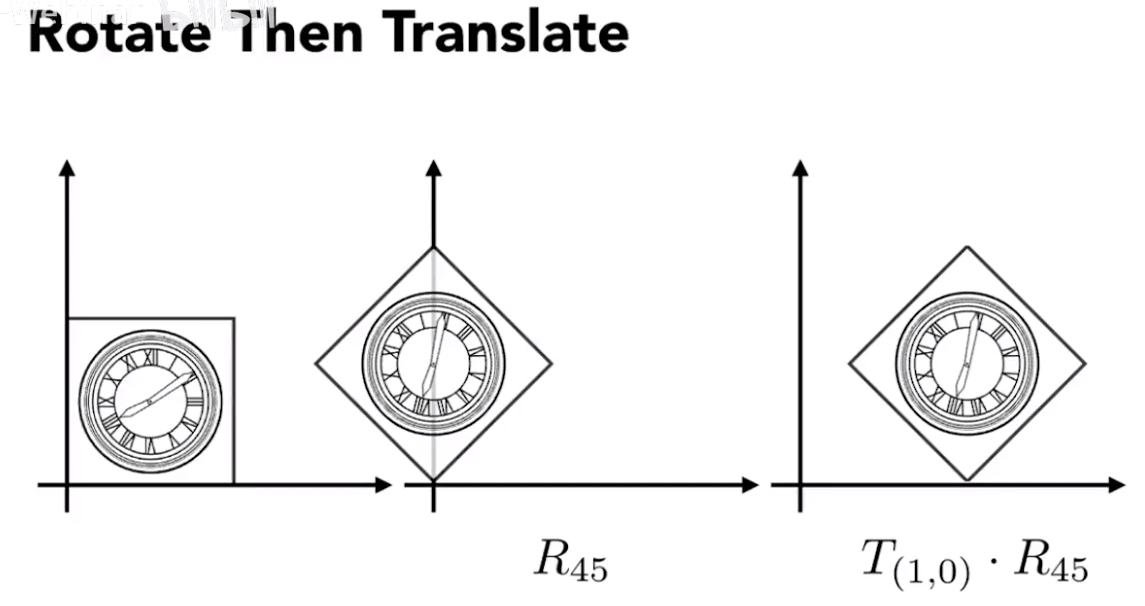$R_{45} \cdot T_{(1,0)} \neq T_{(1,0)} \cdot R_{45}$

$T_{(1,0)} \cdot R_{45}\left[\begin{array}{l}x \\\ y \\\ 1\end{array}\right]=\left[\begin{array}{ccc}1 & 0 & 1 \\\ 0 & 1 & 0 \\\ 0 & 0 & 1\end{array}\right]\left[\begin{array}{ccc}\cos 45^{\circ} & -\sin 45^{\circ} & 0 \\\ \sin 45^{\circ} & \cos 45^{\circ} & 0 \\\ 0 & 0 & 1\end{array}\right]\left[\begin{array}{l}x \\\ y \\\ 1\end{array}\right]$三维变换

$\left(\begin{array}{l}x^{\prime} \\\ y^{\prime} \\\ z^{\prime} \\\ 1\end{array}\right)=\left(\begin{array}{llll}a & b & c & t_{x} \\\ d & e & f & t_{y} \\\ g & h & i & t_{z} \\\ 0 & 0 & 0 & 1\end{array}\right) \cdot\left(\begin{array}{l}x \\\ y \\\ z \\\ 1\end{array}\right)$

Scale

$\mathbf{S}\left(s_{x}, s_{y}, s_{z}\right)=\left(\begin{array}{cccc}s_{x} & 0 & 0 & 0 \\\ 0 & s_{y} & 0 & 0 \\\ 0 & 0 & s_{z} & 0 \\\ 0 & 0 & 0 & 1\end{array}\right)$

Translation

$\mathbf{T}\left(t_{x}, t_{y}, t_{z}\right)=\left(\begin{array}{cccc}1 & 0 & 0 & t_{x} \\\ 0 & 1 & 0 & t_{y} \\\ 0 & 0 & 1 & t_{z} \\\ 0 & 0 & 0 & 1\end{array}\right)$

Rotation

绕轴旋转

Rotation around x-, y-, or z-axis

$\mathbf{R}_{x}(\alpha)=\left(\begin{array}{cccc}1 & 0 & 0 & 0 \\\ 0 & \cos \alpha & -\sin \alpha & 0 \\\ 0 & \sin \alpha & \cos \alpha & 0 \\\ 0 & 0 & 0 & 1\end{array}\right)$

$\mathbf{R}_{y}(\alpha)=\left(\begin{array}{cccc}\cos \alpha & 0 & \sin \alpha & 0 \\\ 0 & 1 & 0 & 0 \\\ -\sin \alpha & 0 & \cos \alpha & 0 \\\ 0 & 0 & 0 & 1\end{array}\right)$

$\mathbf{R}_{z}(\alpha)=\left(\begin{array}{cccc}\cos \alpha & -\sin \alpha & 0 & 0 \\\ \sin \alpha & \cos \alpha & 0 & 0 \\\ 0 & 0 & 1 & 0 \\\ 0 & 0 & 0 & 1\end{array}\right)$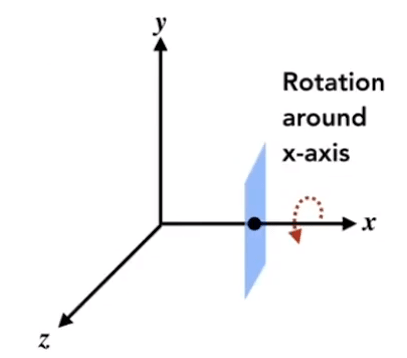Rodrigues’ Rotation FormulaRotation by angle α round axis n

$\mathbf{R}(\mathbf{n}, \alpha)=\cos (\alpha) \mathbf{I}+(1-\cos (\alpha)) \mathbf{n} \mathbf{n}^{T}+\sin (\alpha)\left(\begin{array}{ccc}0 & -n_{z} & n_{y} \\\ n_{z} & 0 & -n_{x} \\\ -n_{y} & n_{x} & 0\end{array}\right)$

GAMES101_Lecture_04_supp.pdf

View/Camera Transformation 视图变换

定义相机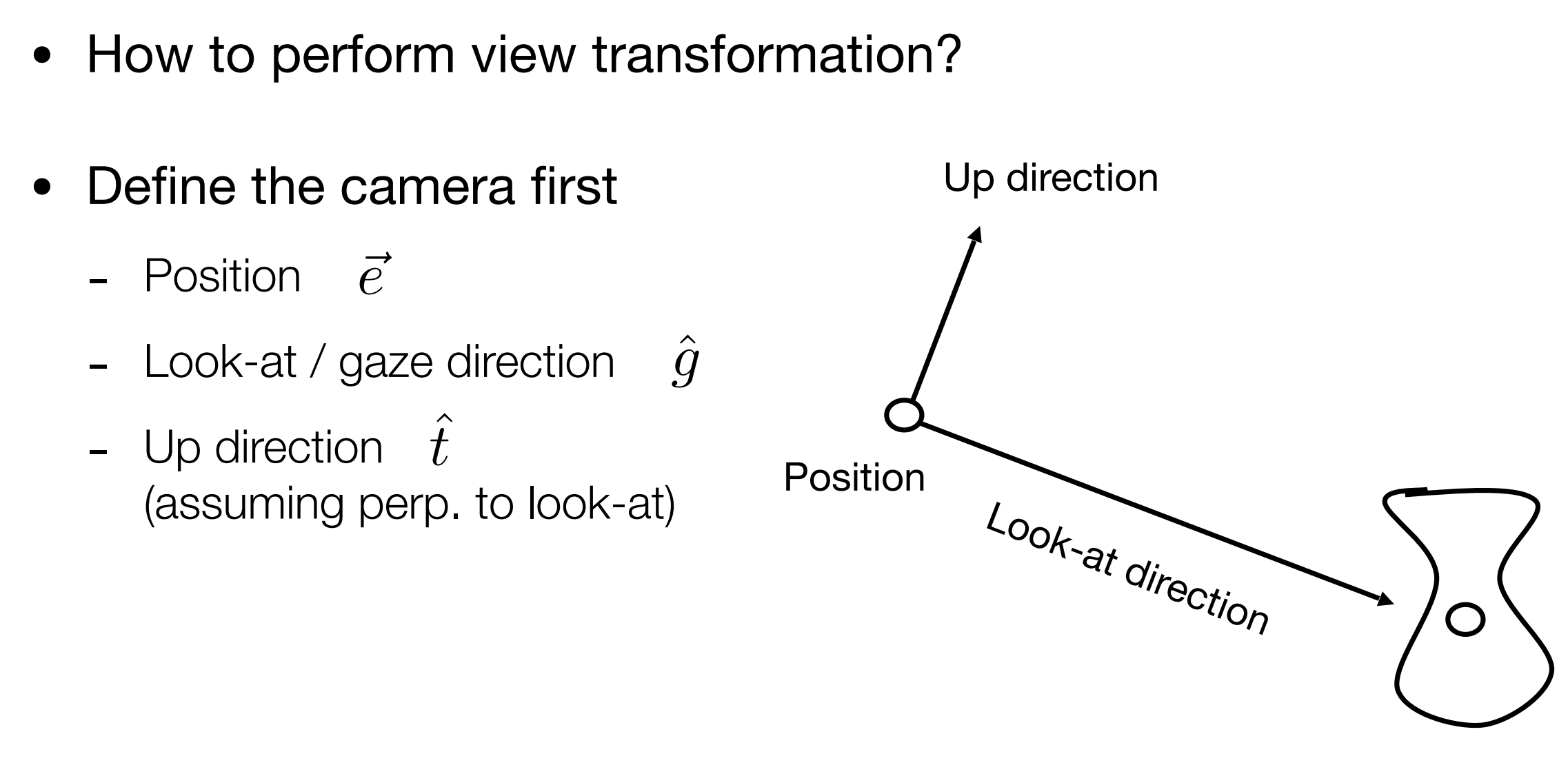视图变换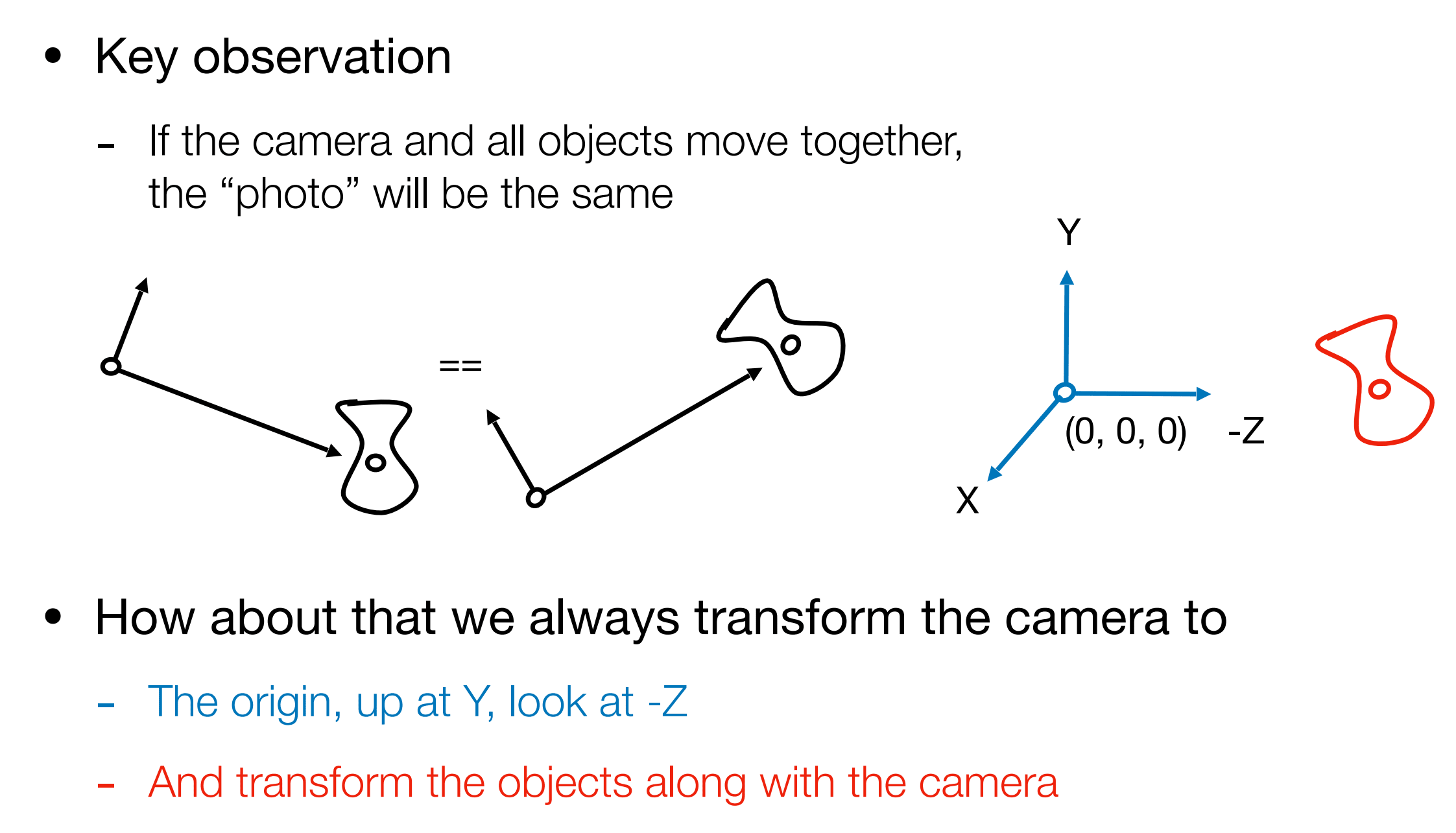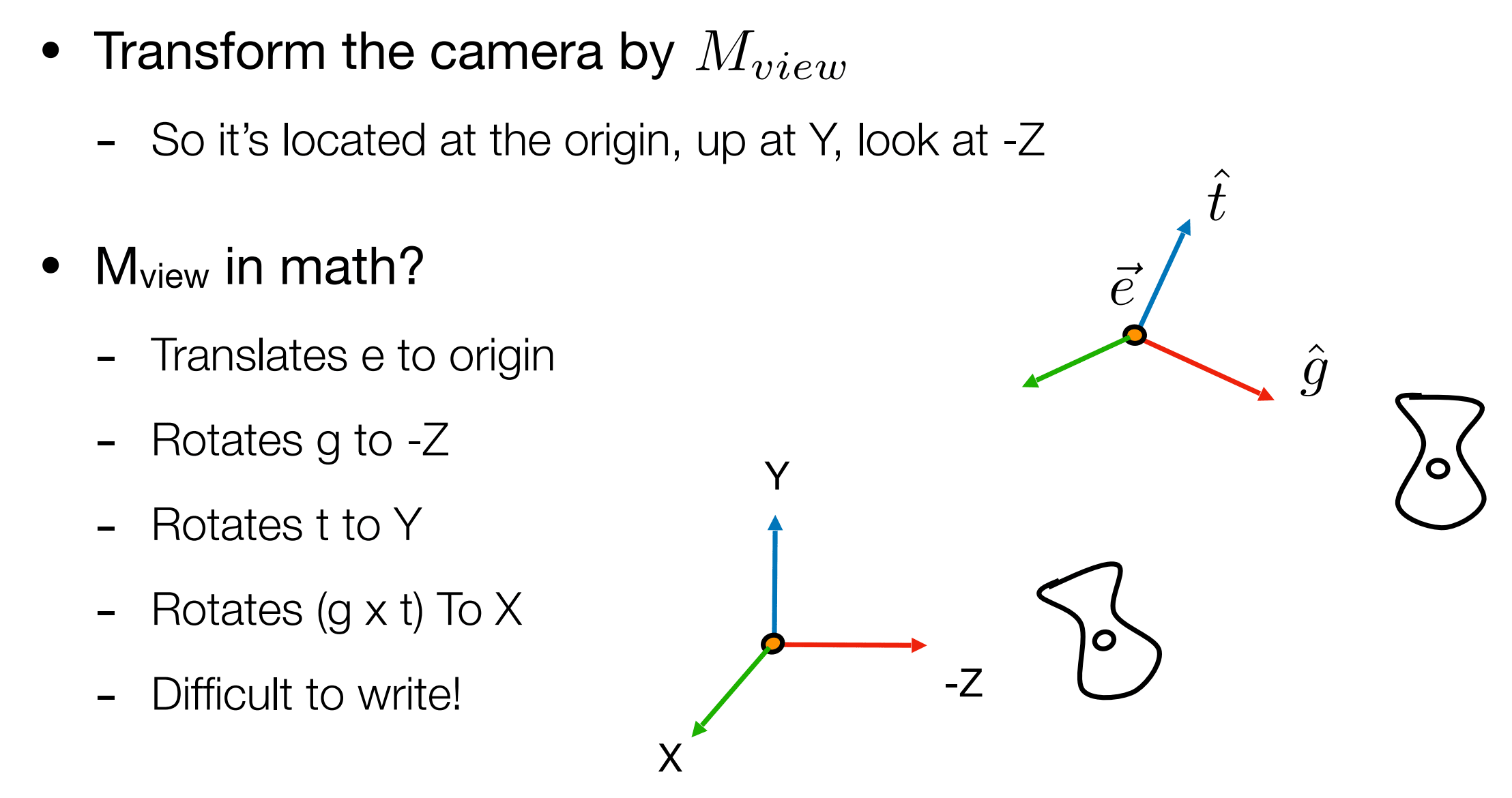求视图变换矩阵

1. 先把相机中心 e 平移到原点

$T_{v i e w}=\left[\begin{array}{cccc}1 & 0 & 0 & -x_{e} \\\ 0 & 1 & 0 & -y_{e} \\\ 0 & 0 & 1 & -z_{e} \\\ 0 & 0 & 0 & 1\end{array}\right]$

1. 把观察的方向 g 旋转到 -z 上，把向上方向 t 旋转到 y 方向上，g X t (g 叉乘 t)的方向旋转到 x 方向上
• Rotate g to -z , t to y, g X t To x （世界空间到观察空间）
• Consider its inverse rotation: x to g X t , y to t, z to -g （观察空间到世界空间）

x to g X t , y to t, z to -g 的旋转矩阵就是：

$R_{v i e w}^{-1}=\left[\begin{array}{cccc}x_{\hat{g} \times \hat{t}} & x_{t} & x_{-g} & 0 \\\ y_{\hat{g} \times \hat{t}} & y_{t} & y_{-g} & 0 \\\ z_{\hat{g} \times \hat{t}} & z_{t} & z_{-g} & 0 \\\ 0 & 0 & 0 & 1\end{array}\right]$$R_{v i e w}=\left[\begin{array}{cccc}x_{\hat{g} \times \hat{t}} & y_{\hat{g} \times \hat{t}} & z_{\hat{g} \times \hat{t}} & 0 \\\ x_{t} & y_{t} & z_{t} & 0 \\\ x_{-g} & y_{-g} & z_{-g} & 0 \\\ 0 & 0 & 0 & 1\end{array}\right]$Projection Transformation 投影变换

Projection in Computer Graphics

• 3D to 2D
• Orthographic projection
• Perspective projectionPerspective projection vs. orthographic projection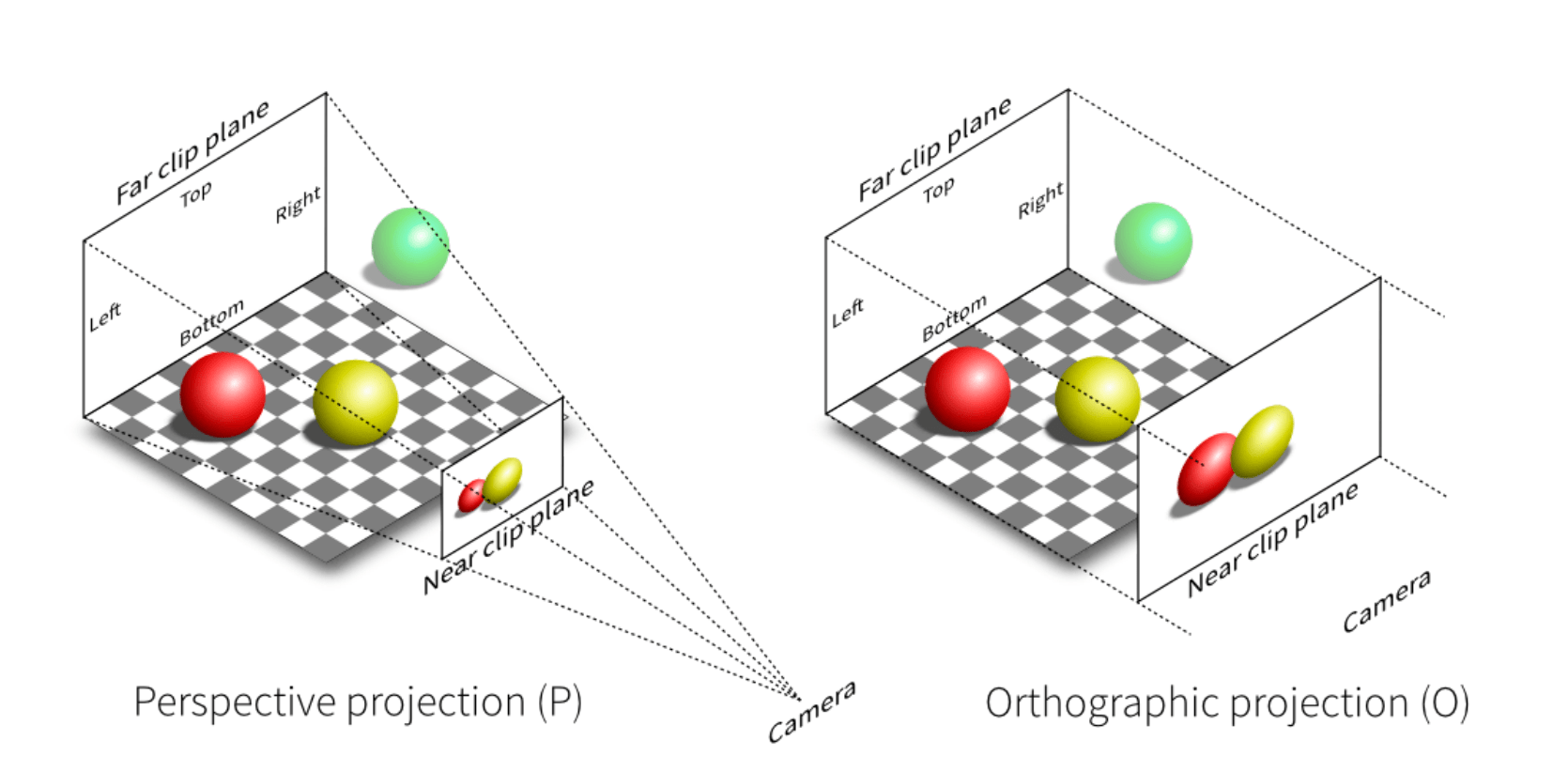Orthographic Projection 正交投影

方法一

A simple way of understanding

• Camera located at origin, looking at -Z, up at Y (looks familiar?)
• Drop Z coordinate
• Translate and scale the resulting rectangle to $[-1,1]^{2}$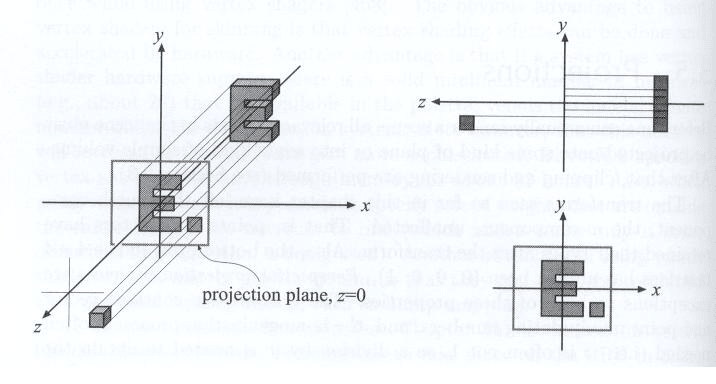方法二

In general, we want to map a cuboid [l, r] x [b, t] x [f, n] to the “canonical (正则、规范、标准)” cube [-1,1]^3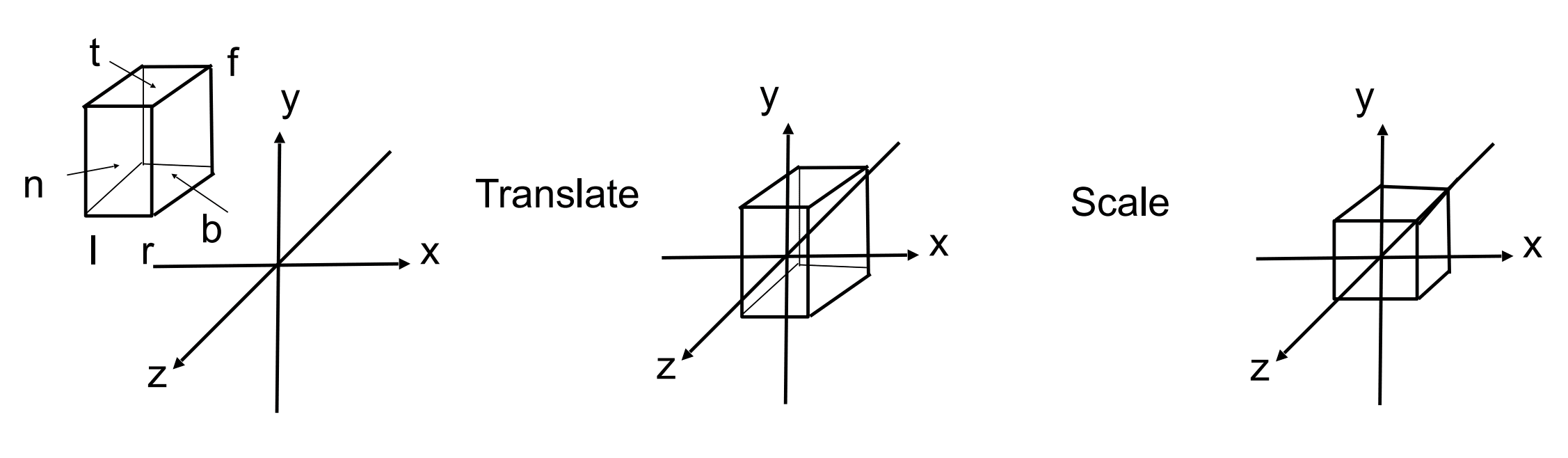Slightly different orders (to the “simple way”)

• Center cuboid by translating 移到原点
• Scale into “canonical” cube 映射到 [-1, 1]，也就是缩放

Translate (center to origin) first, then scale (length/width/height to 2) 因为 -1 到 1 的长度就是 2。Perspective Projection 透视投影

• Most common in Computer Graphics, art, visual system
• Further objects are smaller
• Parallel lines not parallel; converge to single point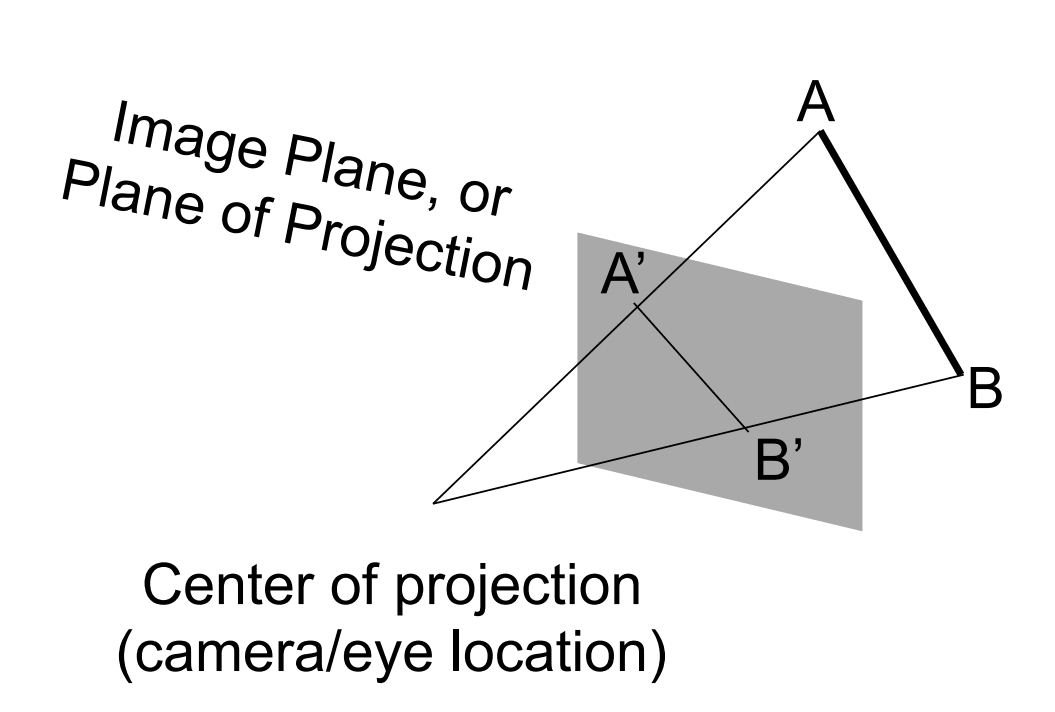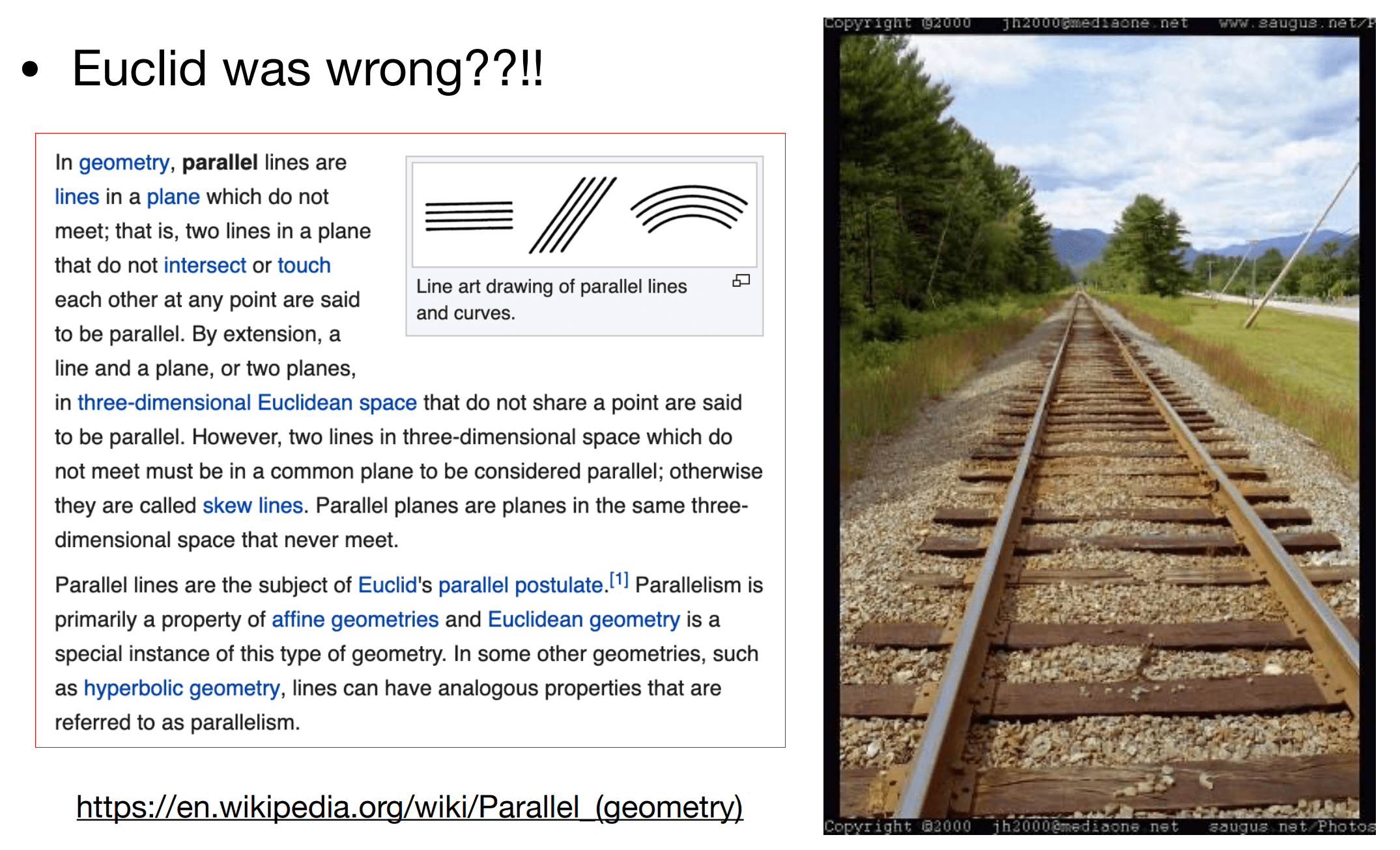Recall

• Before we move on
• Recall: property of homogeneous coordinates
• (x, y, z, 1),(k x, k y, k z, k !=0), (x z, y z, z^2, z !=0) all represent the same point (x, y, z) in 3D
• 只要一个点乘于一个不为零的 k，那么它们还是一个点。那么我们还可以将其乘以 z，其表示的点还是空间中同样的点。下面我们会用到。
• e.g. (1, 0, 0, 1) and (2, 0, 0, 2) both represent (1, 0, 0)
• Simple, but useful

怎么做透视投影

How to do perspective projection

• First “squish” the frustum into a cuboid (n→n, f→f) (M_persp→ortho)
• Do orthographic projection ( M_ortho, already known!)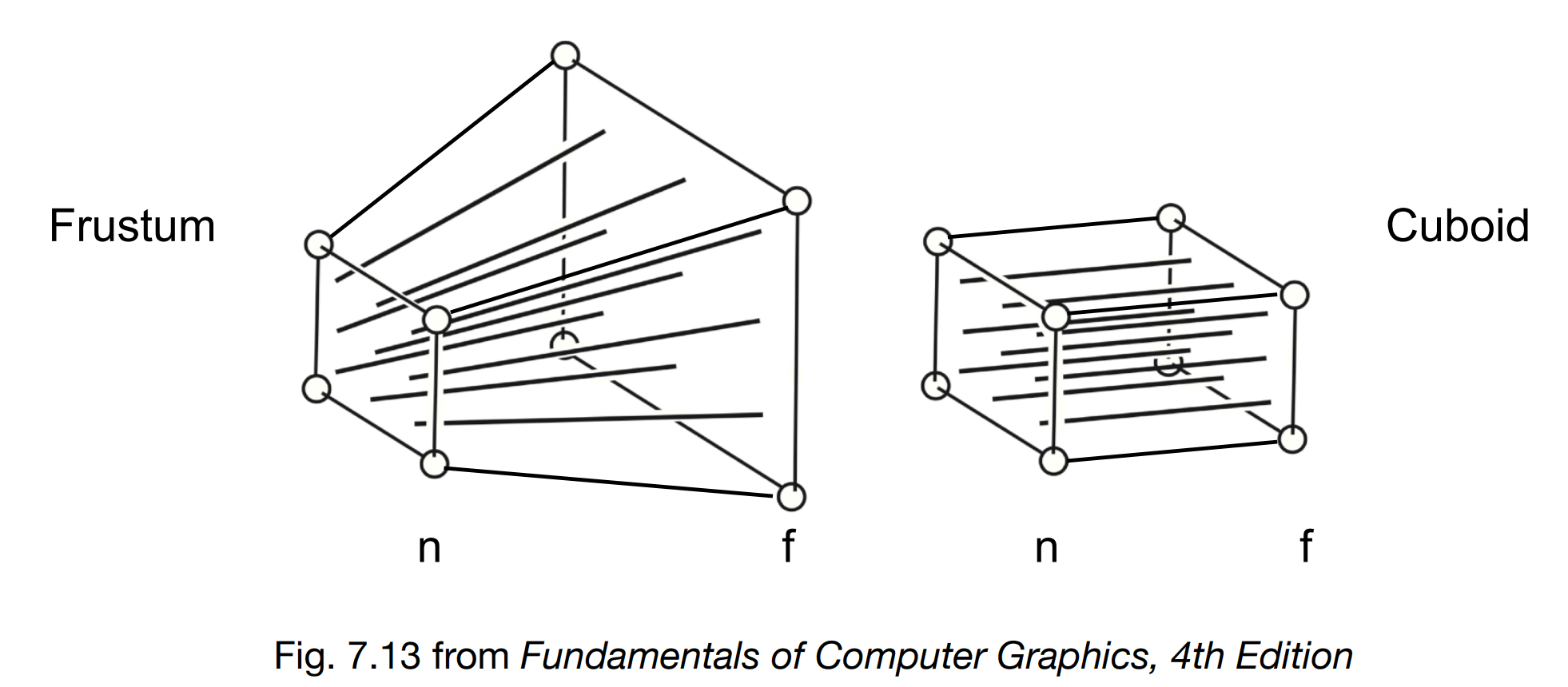• 近平面上任何一个点不变。
• Z 值不变
• 远平面的中心也不会发生变化

求出任何一个点挤压后的 x’, y’ 值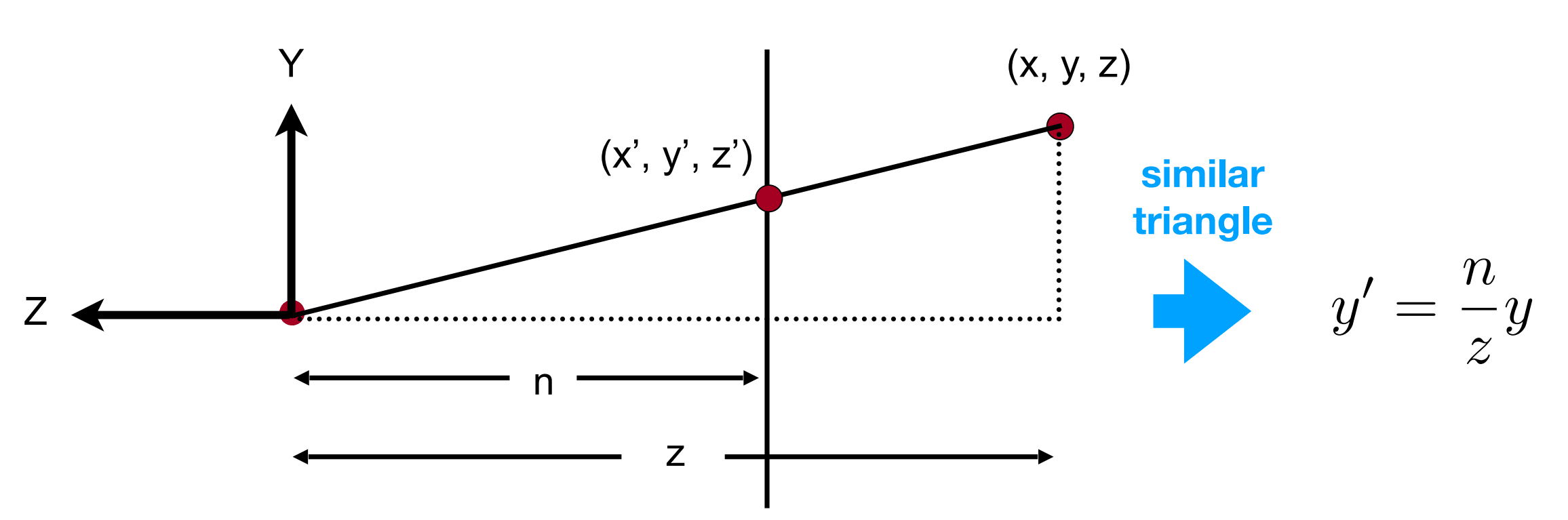$y^{\prime}=\frac{n}{z} y$

$x^{\prime}=\frac{n}{z} x$$M_{p e r s p \rightarrow o r t h o}^{(4 \times 4)}\left(\begin{array}{c}x \\\ y \\\ z \\\ 1\end{array}\right)=\left(\begin{array}{c}n x \\\ n y \\\ \text { unknown } \\\ z\end{array}\right)$

$M_{p e r s p \rightarrow o r t h o}=\left(\begin{array}{llll}n & 0 & 0 & 0 \\\ 0 & n & 0 & 0 \\\ ? & ? & ? & ? \\\ 0 & 0 & 1 & 0\end{array}\right)$

Observation: the third row is responsible for z’

• Any point on the near plane will not change
• 近平面的点不变，对于任何 (x,y,n,1) 运算完了一定还是 (x,y,n,1)
• Any point’s z on the far plane will not change
• 远平面的点，虽然 x, y 会变化，但是 z 没有变。

求出任何一个点挤压后的 z’ 值$A n+B=n^{2}$Depth Precision Visualized

定义视锥

vertical field-of-view (fovY) and aspect ratioHow to convert from fovY and aspect to l, r, b, t?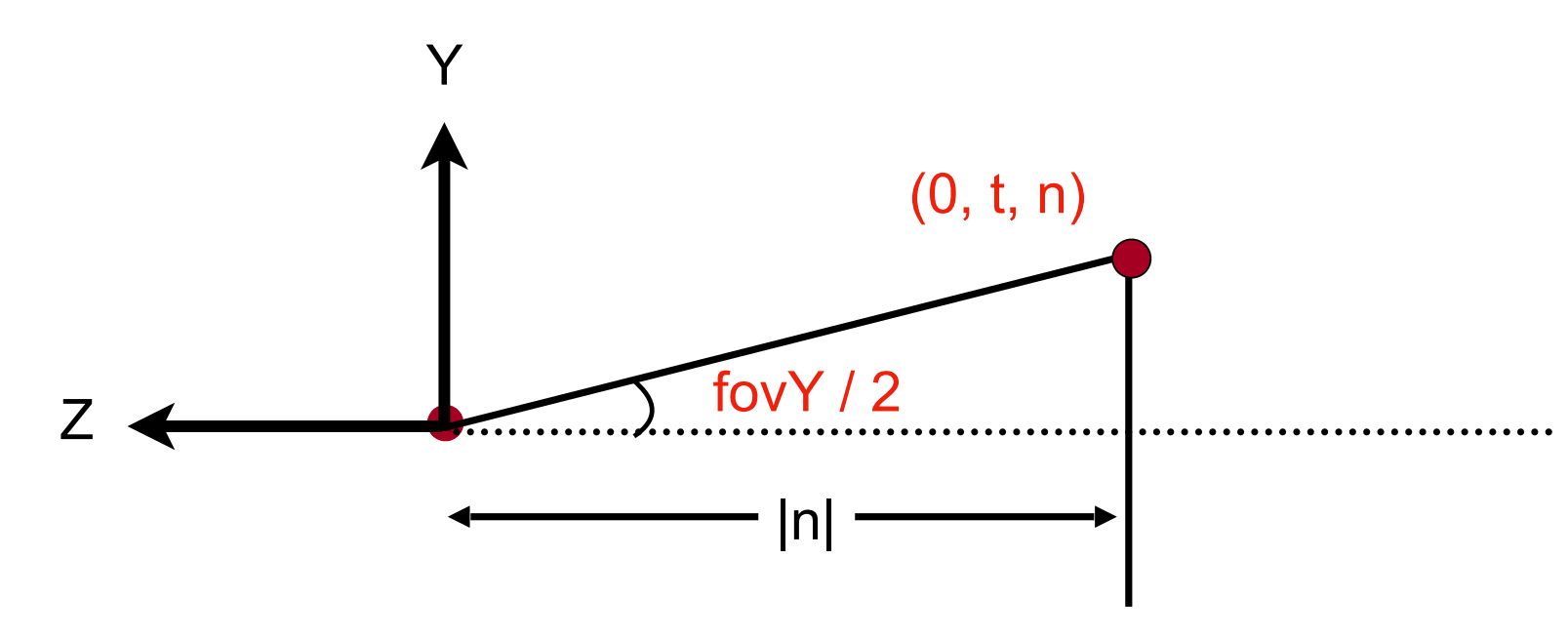完成推导正交投影矩阵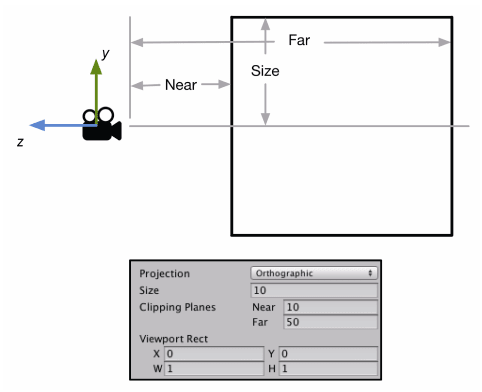Size 属性用来更改视锥体竖直方向上高度的一半，也就是前面近平面的高度 t。

$Aspect\cdot \text{近远平面的高度}=2\cdot Aspect\cdot Size=2\cdot Aspect\cdot t$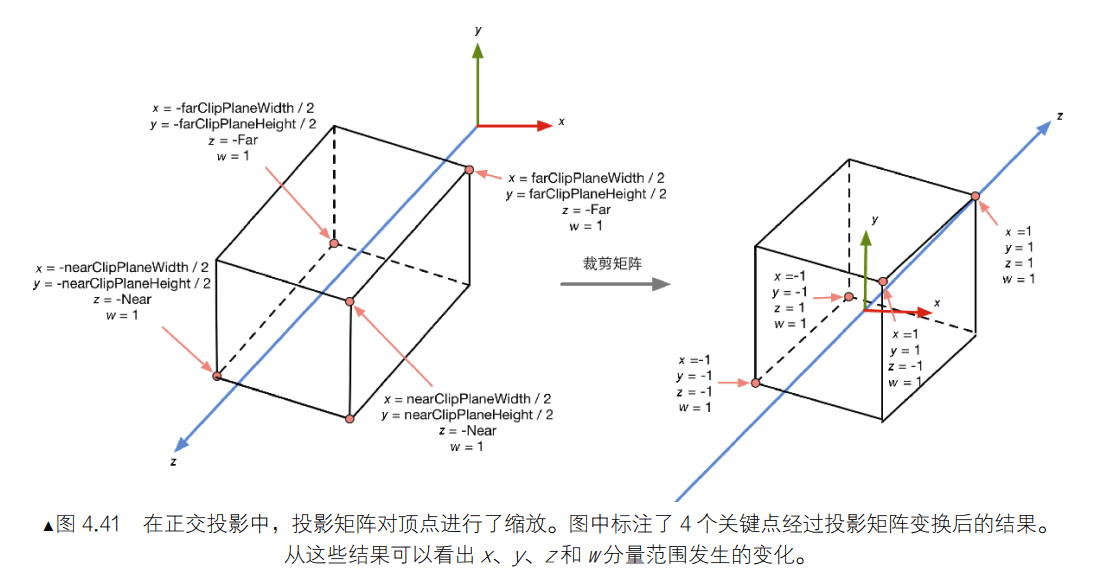完成推导透视投影矩阵